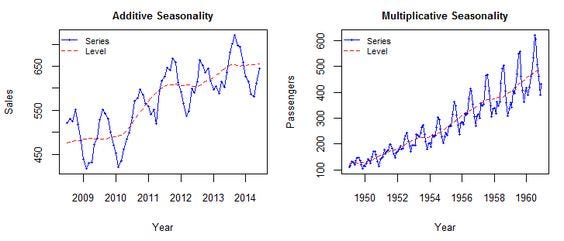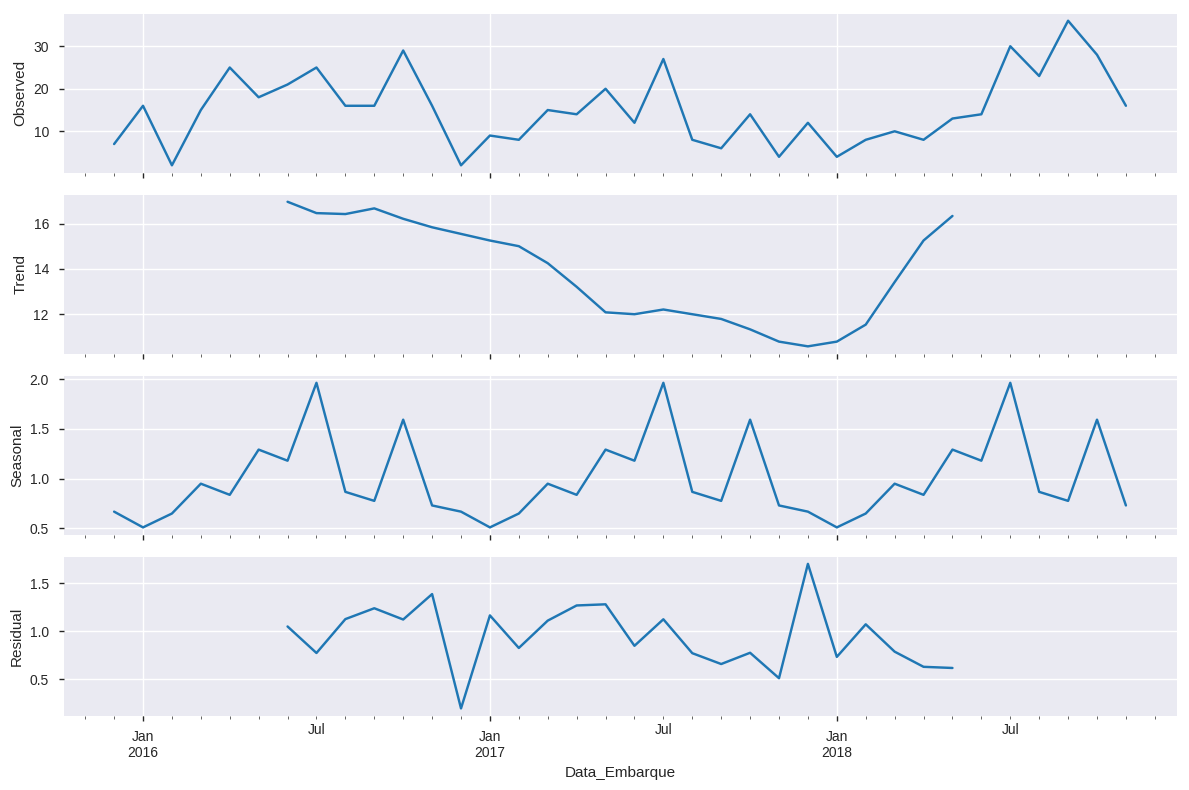# Seasonality in Python: additive or multiplicative model?

Have you started analyzing your charts and realized that more than half of them have varied over time? Congratulations, it seems you have a Time Series in your hands :)

Working with Time Series is not so complicated when we have in hand powerful “features” in Python like Pandas and the Statsmodels library.
These tools make our lives much easier as Data Scientists.

But, look, it’s not a seven-headed creature. However, do not forget to make sure that it is a sequence taken at equal successive spaced points in time. And if you are using Pandas, for example, to index your date frame by date field and also check if the data type of this field is actually date and not just object type, OK?

An important feature to be analyzed in a Time Series is the seasonality. This is the characteristic of a Time Series having a default behavior within a certain time interval. If this pattern is repeated within the same interval over time then we will have the presence of a sample with a seasonal behavior.
The Statsmodels library helps us perform this analysis with great ease, but first, let’s understand what the analysis features of a Time Series are.

• Level: is the average value in the series.
• Trend: is the increasing or decreasing value in the series.
• Seasonality: is the repeating the short-term cycle in the series.
• Noise: is the random variation in the series.

There are basically two methods to analyze the seasonality of a Time Series: additive and multiplicative.

# The Additive Model

Synthetically it is a model of data in which the effects of the individual factors are differentiated and added to model the data. It can be represented by:

`y(t) = Level + Trend + Seasonality + Noise`

In the additive model, the behavior is linear where changes over time are consistently made by the same amount, like a linear trend. In this situation, the linear seasonality has the same amplitude and frequency.

# The Multiplicative Model

In this situation, trend and seasonal components are multiplied and then added to the error component. It is not linear, can be exponential or quadratic and represented by a curved line as below:

`y(t) = Level * Trend * Seasonality * Noise`

Different from the additive model, the multiplicative model has an increasing or decreasing amplitude and/or frequency over time.

# Additive or multiplicative?

These charts can summarize much of this article. Note that the additive model does not vary in frequency and amplitude over time. The multiplicative model does, in this second model, the behavior acts as an increasing funnel (which may be decreasing) ;)Fig 1 - Additive or Multiplicative Seasonality. Source: https://goo.gl/szKmgo

So, how you should have noticed, we use multiplicative models when the magnitude of the seasonal pattern in the data depends on the magnitude of the data. On other hand, in the additive model, the magnitude of seasonality does not change in relation to time.

# Let’s play

Using Python and Pandas, let’s first prepare our data. Understanding that we have a Data Frame we will reset our index and then set an index based on a date field. Use the command df.dtypes to check the data types.

`import pandas as pddf.reset_index(inplace=True)df['Date'] = pd.to_datetime(df['Date'])df = df.set_index('Date')`

You can also do that and create a new Data Frame with the indexed data.

`df_agg = df_agg.set_index('Date')df_agg.index`

Here, I ‘grouped’ the data by date by summing the Processes by Date values. It works like SQL Group By.

`df_agg = df.groupby('Date')['Process'].sum()`

In order to ensure that my time series has the same distance between the timepoints I use the resample feature here. This is method for frequency conversion and resampling of time series. In that case I’m using a M - monthly resample.

`y = df_agg[‘Process’].resample(‘M’).sum()`

Now comes the magic of Statsmodels. Just plot the decomposition of your Data Frame and plim, this beautiful series of charts appears. Just for comparison, I plotted the two methods, first the additive and then the multiplicative. Notice that in the seasonality subplot there is difference on the Y axis but the behavior is the same.

`import statsmodels.api as smdecomposition = sm.tsa.seasonal_decompose(y, model=’additive’)decomposition.plot()`
`import statsmodels.api as smdecomposition = sm.tsa.seasonal_decompose(y, model=’multiplicative’)decomposition.plot()`Fig 3 — Multiplicative Model Example

It is also possible to access the components using these commands:

`decomposition.residdecomposition.seasonaldecomposition.trenddecomposition.observed`

# Conclusions

Analyzing chart seasonality is fun and easy when we use the right tools and we know how to “read the chart”. Time series is a fantastic area and can yield great analysis in Data Science.
Before choosing between Additive or Multiplicative models, take a good look at the behavior of your chart. My suggestion is to make a resample using different ranges and take a look.
In addition to these two methods of seasonal analysis there is the combined model, however (as far as I know) not yet supported by Statsmodels.

If you’re in a hurry, see the title of this article, the first paragraph, and figure 1. That should help you understand;)

Good Forecasts!

--

--